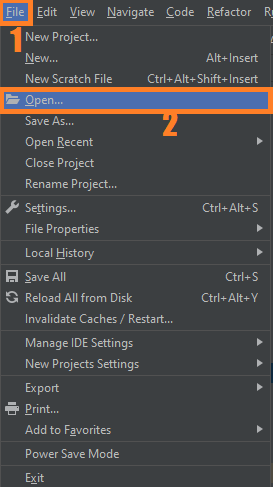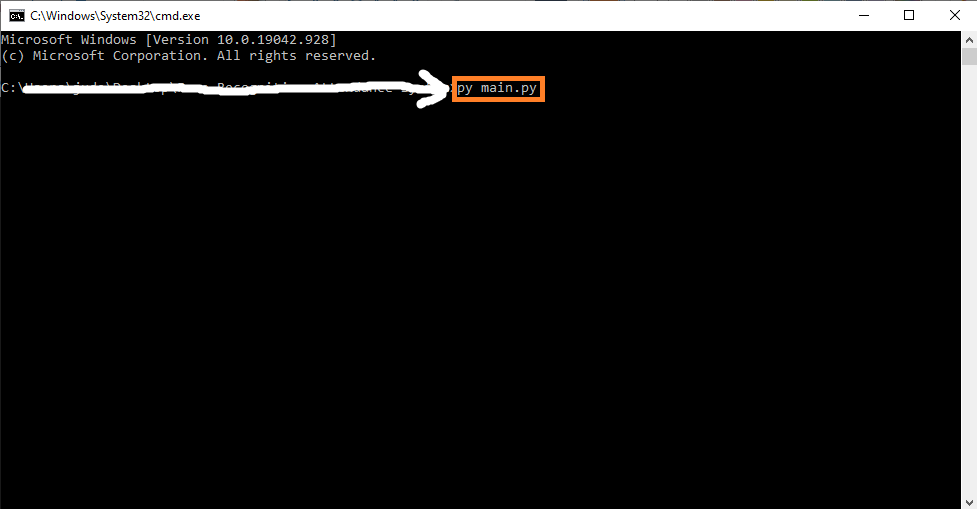# Handwritten Digit Recognition In Python With Source Code

## Handwritten Digit Recognition In Python With Source Code

The Handwritten Digit Recognition In Python was developed using Python Deep Learning, This we are going to implement a handwritten digit recognition app using the MNIST dataset. We will be using a special type of deep neural network that is Convolutional Neural Networks. In the end, we are going to build a GUI in which you can draw the digit and recognize it straight away.

A Handwritten Digit Recognition s the ability of computers to recognize human handwritten digits. It is a hard task for the machine because handwritten digits are not perfect and can be made with many different flavors. The handwritten digit recognition is the solution to this problem which uses the image of a digit and recognizes the digit present in the image.

By the way, if you are new to python programming and you don’t know what would be the Python IDE to use, I have here a list of the Best Python IDE for Windows, Linux, Mac OS that will suit you. I also have here How to Download and Install the Latest Version of Python on Windows.

To start executing Handwritten Digit Recognition In Python With Source Code, make sure that you have installed Python 3.9 and PyCharm on your computer.

## Handwritten Digit Recognition In Python With Source Code : Steps on how to run the project.

Time needed: 5 minutes.

These are the steps on how to run Handwritten Digit Recognition In Python With Source Code

First, download the given source code below and unzip the source code.• Step 2: Import the project to your PyCharm IDE.• Step 3: Run the project.

last, run the project with the command “py main.py”## Installed Libraries

```from keras.models import load_model
from tkinter import *
import tkinter as tk
import win32gui
from PIL import ImageGrab, Image
import numpy as np```

## Complete Source Code

```from keras.models import load_model
from tkinter import *
import tkinter as tk
import win32gui
from PIL import ImageGrab, Image
import numpy as np

def predict_digit(img):
#resize image to 28x28 pixels
img = img.resize((28,28))
#convert rgb to grayscale
img = img.convert('L')
img = np.array(img)
#reshaping to support our model input and normalizing
img = img.reshape(1,28,28,1)
img = img/255.0
#predicting the class
res = model.predict([img])
return np.argmax(res), max(res)

class App(tk.Tk):
def __init__(self):
tk.Tk.__init__(self)

self.x = self.y = 0

# Creating elements
self.canvas = tk.Canvas(self, width=300, height=300, bg = "white", cursor="cross")
self.label = tk.Label(self, text="Draw..", font=("Helvetica", 48))
self.classify_btn = tk.Button(self, text = "Recognise", command = self.classify_handwriting)
self.button_clear = tk.Button(self, text = "Clear", command = self.clear_all)

# Grid structure

#self.canvas.bind("<Motion>", self.start_pos)
self.canvas.bind("<B1-Motion>", self.draw_lines)

def clear_all(self):
self.canvas.delete("all")

def classify_handwriting(self):
HWND = self.canvas.winfo_id()  # get the handle of the canvas
rect = win32gui.GetWindowRect(HWND)  # get the coordinate of the canvas
a,b,c,d = rect
rect=(a+4,b+4,c-4,d-4)
im = ImageGrab.grab(rect)

digit, acc = predict_digit(im)
self.label.configure(text= str(digit)+', '+ str(int(acc*100))+'%')

def draw_lines(self, event):
self.x = event.x
self.y = event.y
r=8
self.canvas.create_oval(self.x-r, self.y-r, self.x + r, self.y + r, fill='black')

app = App()
mainloop()
```

## Summary

In this article, we have successfully built a Python deep learning project on handwritten digit recognition app. We have built and trained the Convolutional neural network which is very effective for image classification purposes. Later on, we build the GUI where we draw a digit on the canvas then we classify the digit and show the results.

## Inquiries

1.2.